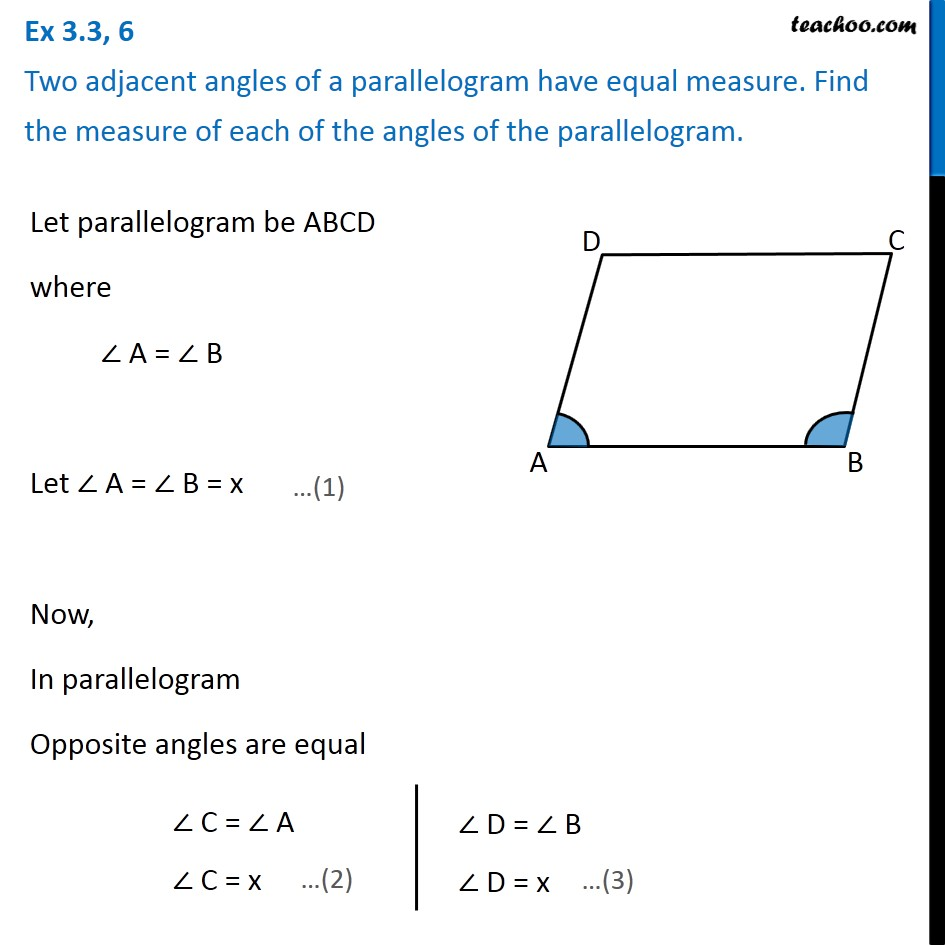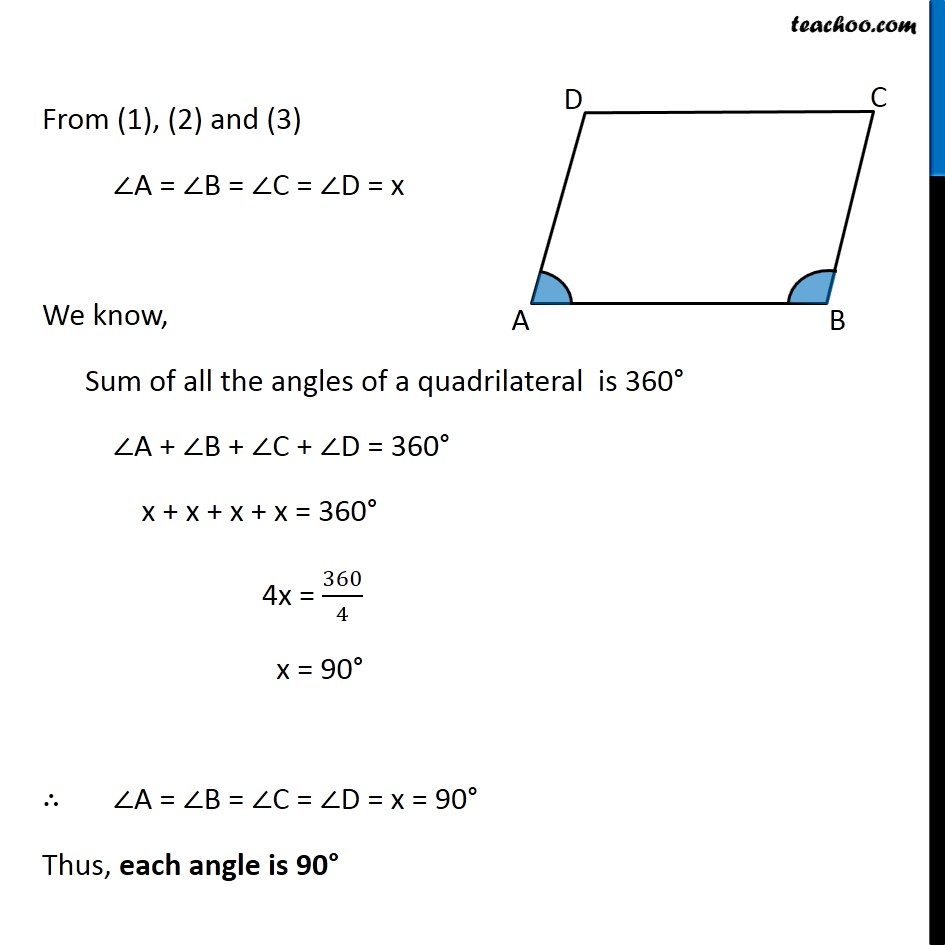Angles of Parallelogram

Chapter 3 Class 8 Understanding Quadrilaterals
Concept wiseIntroducing your new favourite teacher - Teachoo Black, at only ₹83 per month

### Transcript

Ex 3.3, 6 Two adjacent angles of a parallelogram have equal measure. Find the measure of each of the angles of the parallelogram.Let parallelogram be ABCD where ∠ A = ∠ B Let ∠ A = ∠ B = x Now, In parallelogram Opposite angles are equal ∠ C = ∠ A ∠ C = x ∠ D = ∠ B ∠ D = x From (1), (2) and (3) ∠A = ∠B = ∠C = ∠D = x We know, Sum of all the angles of a quadrilateral is 360° ∠A + ∠B + ∠C + ∠D = 360° x + x + x + x = 360° 4x = 360/4 x = 90° ∴ ∠A = ∠B = ∠C = ∠D = x = 90° Thus, each angle is 90°